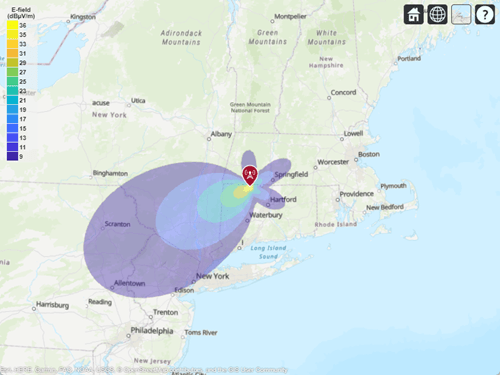# Visualize Antenna Field Strength Map on Earth

This example shows how to calculate an antenna's field strength on flat earth and display it on a map.

### Create Helix

Use the default helix, and rotate it by 90 degrees so that most of its field is along the XY-plane, i.e. on the Earth's surface.

```f0 = 2.4e9; ant = helix('Tilt',-90); f = figure; show(ant) view(-220,30)```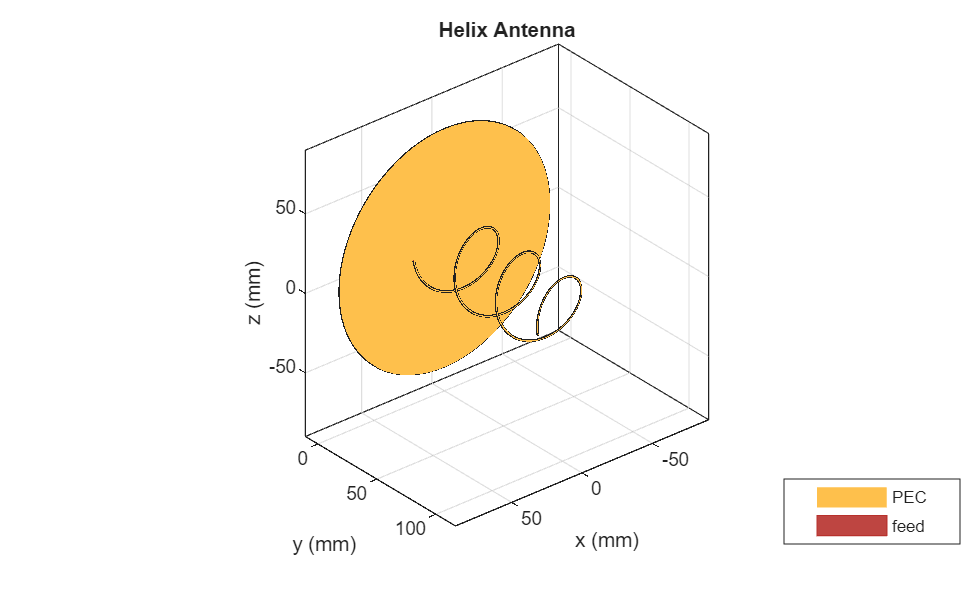### Calculate the Electric Field at Various Points in Space

Assume the origin of the tilted antenna is located 15 m above the ground. Calculate the electric field in a rectangular region covering 500-by-500 km.

```z = -15; x = (-250:4:250)*1e3; y = (-100:4:400)*1e3; [X,Y] = meshgrid(x,y); numpoints = length(x)*length(y); points = [X(:)'; Y(:)'; z*ones(1,numel(X))]; E = EHfields(ant,f0,points); % Units: V/m```

### Calculate the Magnitude of Electric Field

Compute the magnitude of the electric field.

```Emag = zeros(1,numpoints); for m=1:numpoints Emag(m) = norm(E(:,m)/sqrt(2)); end Emag = 20*log10(reshape(Emag,length(y),length(x))); % Units: dBV/m Emag = Emag + 120; % Units: dBuV/m```

### Plot the Electric Field

Plot the magnitude of the electric field as a function of the x and y distance.

```d_min = min(Emag(:)); d_max = max(Emag(:)); del = (d_max-d_min)/12; d_vec = round(d_min:del:d_max); if isvalid(f) close(f) end figure contourf(X*1e-3,Y*1e-3,Emag,d_vec,'showtext','on') title('Field Strength (dB\muV/m) on flat Earth (1V tx)') xlabel('lateral (km)') ylabel('boresight (km)') c = colorbar; set(get(c,'title'),'string','dB\muV/m')```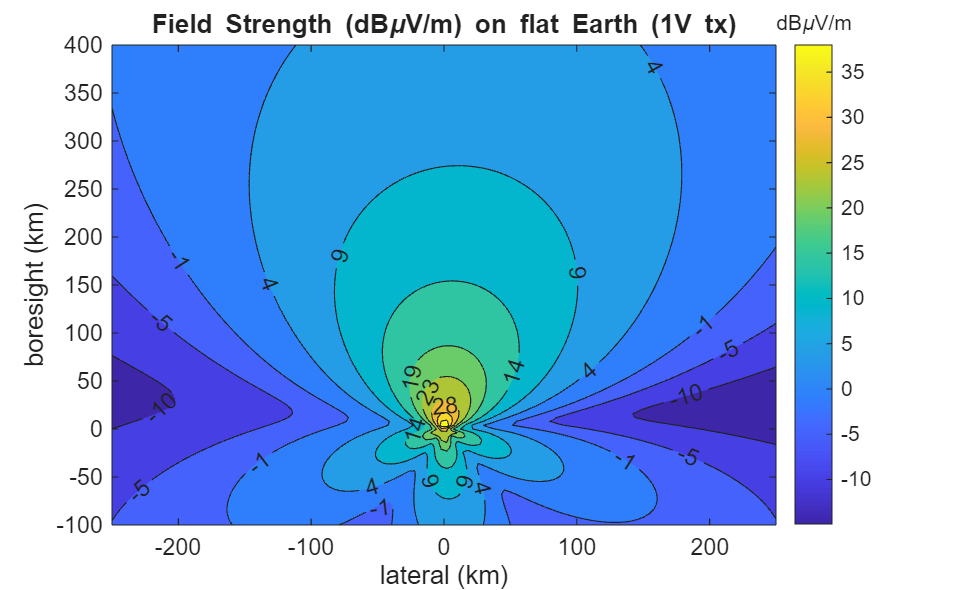### Define Transmitter Site with Antenna

Specify location and orientation of the antenna. Assign a location of 42 degrees north and 73 degrees west, along with antenna height of 15 meters. Orient the antenna toward the southwest at an angle of -150 degrees (or, equivalently, +210 degrees) measured counterclockwise from east.

```lat = 42; lon = -73; h = 15; az = -150;```

Because the antenna points along the local Y-axis (not the X-axis), subtract 90 degrees to determine the rotation of the local system with respect to xEast-yNorth. (This is the angle to the local X-axis from xEast measured counterclockwise.)

`xyrot = wrapTo180(az - 90);`

Define a transmitter site with the antenna and orientation defined above.

```tx = txsite('Name','Antenna Site', ... 'Latitude',lat, ... 'Longitude',lon, ... 'Antenna',ant, ... 'AntennaHeight',h, ... 'AntennaAngle',xyrot, ... 'TransmitterFrequency',f0);```

### Calculate Transmitter Power

Calculate root mean square value of power corresponding to antenna EHfields calculation and set as transmitter power.

```Z = impedance(tx.Antenna,tx.TransmitterFrequency); If = feedCurrent(tx.Antenna,tx.TransmitterFrequency); Irms = norm(If)/sqrt(2); Ptx = real(Z)*(Irms)^2; tx.TransmitterPower = Ptx;```

### Display Electric Field Coverage Map with Single Contour

Show transmitter site marker on a map and display coverage zone. Specify a signal strength of 9 dBuV/m to display a single electric field contour. Specify the propagation model as 'freespace' to model idealized wave propagation in free space, which disregards obstacles due to curvature of the Earth or terrain.

```% Launch Site Viewer with no terrain viewer = siteviewer("Terrain", "none"); % Plot coverage coverage(tx,'freespace', ... 'Type','efield', ... 'SignalStrengths',9)```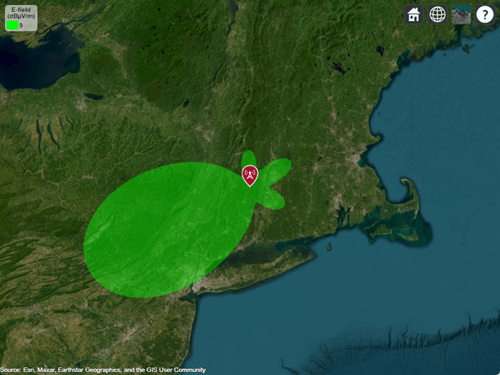### Customize Site Viewer

Set the map imagery using the `Basemap` property. Alternatively, open the map imagery picker in Site Viewer by clicking the second button from the right. Select "Topographic" to see topography and labels on the map.

`viewer.Basemap = 'topographic'; `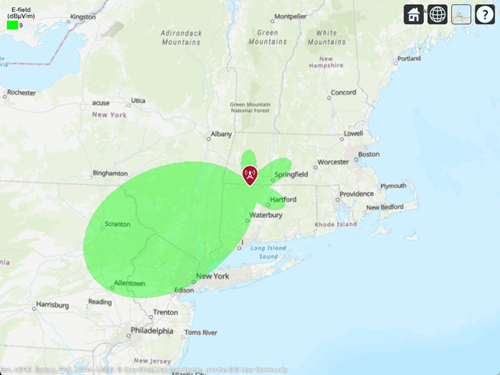### Display Electric Field Coverage Map with Multiple Contours

Specify multiple signal strengths to display multiple electric field contours.

```sigStrengths = [9 14 19 24 29 36]; coverage(tx,'freespace', ... 'Type','efield', ... 'SignalStrengths',sigStrengths, ... 'Colormap','parula', ... 'ColorLimits',[9 36])```